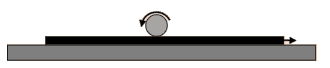# Cylinder on a moving board

• Mark128
In summary, the conversation is about solving a physics problem involving conservation of momentum and angular momentum. The person has tried solving it by taking the center of the cylinder as the axis, but there is friction between the cylinder and the board which causes a change in angular momentum. They suggest taking moments about the point of contact as an alternative solution.f

#### Mark128

Homework Statement
On the flat surface lays a flat board with mass M, and on the board - a cylinder with mass m and radius r. Initially, board moves with velocity v, and cylinder rotates with such angular velocity, that it's stationary relative to the ground. Because of friciton between the board and surface, the board stops after some time. What is velocity of cylinder after that happens?
Relevant Equations
--I've been trying to solve it mainly through conservation of momentum and angular momentum, appareantly it's not correct. My idea was:
I, Moment of inertia = 0.5*m*r^2
L1, initial angular momentum = I * v/r

After a board stops, the change in it's momentum is M*v, so the impulse of torque on the cylinder will be M*v*r.

So the change in angular momentum is M*v*r.

So L2-0.5*m*r^2 = M*v*r

L2 = 0.5*m*r*v_final

So v_final = v*(0.5*m + M)/(0.5*m)

But as mentioned earlier, it's incorrect and I'm also not entirely sure which part is wrong.

•Delta2
You can only take angular momentum about some chosen axis to be conserved in a system if there are no external forces that have a torque about that axis. You appear to be taking the centre of the cylinder as axis, but there is friction (rolling contact) between the cylinder and the board.
What axis could you use to avoid this difficulty?

You can only take angular momentum about some chosen axis to be conserved in a system if there are no external forces that have a torque about that axis. You appear to be taking the centre of the cylinder as axis, but there is friction (rolling contact) between the cylinder and the board.
What axis could you use to avoid this difficulty?

Yes, I was taking a center of the cylinder keeping in mind, that there is friction acting on it, and that's why change in angular momentum (L2-L1) is non-zero.

If I choose point of contact as center, the angular momentum is in fact conserved, but I don't know, how this could help.

Yes, I was taking a center of the cylinder keeping in mind, that there is friction acting on it, and that's why change in angular momentum (L2-L1) is non-zero.

If I choose point of contact as center, the angular momentum is in fact conserved, but I don't know, how this could help.
There are two ways you can solve it:
1. Take moments about the cylinder’s centre, but include the torque from friction with the board and write a linear momentum equation also involving the friction. (You will have terms like ##\int F.dt##.)
2. Take moments about a point at the surface of the board. (Better not to think of it as a point fixed to the board or the point of contact over time since in principle that gets you into noninertial frames.) Since angular momentum of the cylinder is conserved about such a point you can get the answer in one equation.